Next: Parameter Identification Up: Modeling of Physical Previous: Resonance in Multifrequency

Contrast Sensitivity of Luminance Fields

Bert W. Rust, ACMD
Holly E. Rushmeier, Scientific Computing Environments Division
Philip A. Sanders, Building and Fire Research Laboratory
Christine D. Piatko, Computer Systems Laboratory

As part of an effort to quantify the effectiveness of lighting in a working environment, this project seeks to compute the response of the human eye to a given two-dimensional luminance field like the test pattern given in Figure 10.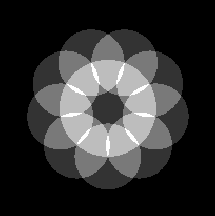Figure 10:  A luminance field test pattern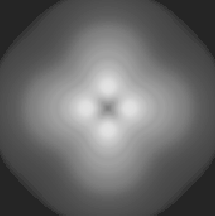Figure 11:  The estimated contrast sensitivity function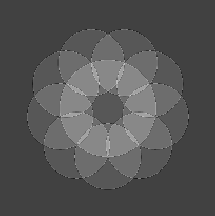Figure 12:  The perceived luminance field

The two-dimensional Fourier amplitude spectrum of the field is multiplied by the contrast sensitivity function of the eye to generate the internal representation which visual psychophysicists believe is the basis for the human percept.

The contrast sensitivity function is based on measured data taken radially in the vertical (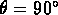) and oblique (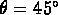) directions. It is assumed that the contrast sensitivity in the horizontal (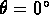) direction is the same as that in the vertical direction, and that the whole function is symmetric about the origin of the frequency space. This suggests a contrast sensitivity function of the form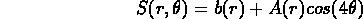where r is the radial distance from the origin (measured in cycles per degree) and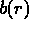and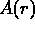are, respectively, a baseline and an amplitude function which must be approximated from the measurements. Discrete approximations of these functions are obtained by averaging and differencing the vertical and oblique measurements. Functional representations are then obtained by fitting optimal smoothing splines to these approximations, with each point weighted inversely by its estimated standard deviation. The smoothing constant in each case was chosen to minimize the generalized cross-validation statistic. A representation of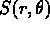is shown in Figure 11 where the horizontal and vertical frequency scales range between -34 and +34 cycles/degree.

Multiplying the Fourier amplitude spectrum of the test pattern in Figure 10 by the contrast sensitivity function in Figure 11 and computing the inverse Fourier transform of the result gives the perceived field shown in Figure 12.Next: Parameter Identification Up: Modeling of Physical Previous: Resonance in Multifrequency